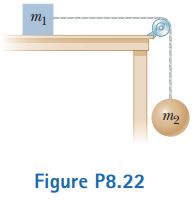# Problem: The coefficient of friction between the block of mass m1 = 3.00 kg and the surface in the figure is µk = 0.400. The system starts from rest. What is the speed of the ball of mass m2 = 5.00 kg when it has fallen a distance h = 1.50 m?

###### FREE Expert Solution
85% (265 ratings)
###### Problem Details

The coefficient of friction between the block of mass m1 = 3.00 kg and the surface in the figure is µk = 0.400. The system starts from rest. What is the speed of the ball of mass m2 = 5.00 kg when it has fallen a distance h = 1.50 m?Frequently Asked Questions

What scientific concept do you need to know in order to solve this problem?

Our tutors have indicated that to solve this problem you will need to apply the Energy in Connected Objects (Systems) concept. You can view video lessons to learn Energy in Connected Objects (Systems). Or if you need more Energy in Connected Objects (Systems) practice, you can also practice Energy in Connected Objects (Systems) practice problems.

How long does this problem take to solve?

Our expert Physics tutor, Julia took 4 minutes and 9 seconds to solve this problem. You can follow their steps in the video explanation above.

What professor is this problem relevant for?

Based on our data, we think this problem is relevant for Professor Jerousek's class at UCF.

What textbook is this problem found in?

Our data indicates that this problem or a close variation was asked in Physics for Scientists and Engineers - Serway Calc 9th Edition. You can also practice Physics for Scientists and Engineers - Serway Calc 9th Edition practice problems.